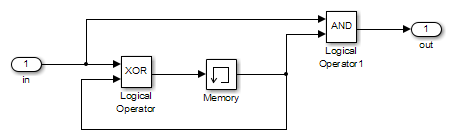## Analyze a Simple ModelThis simple model includes two Logical Operator blocks and a Memory block. The persistent information in this model is limited to the Boolean value of the Memory block. The input to the model is a single Boolean value. The following table describes the complete behavior of the model, including the behavior that results from an arbitrarily long sequence of inputs.

#InputMemory ValueOutput of XOR Block = Next Memory ValueOutput of AND Block
1`false``false``false``false`
2`true``false``true``false`
3`false``true``true``false`
4`true``true``false``true`

The test objective is to generate test cases that result in a `true` output. A `true` output results when the input is `true`, and the output of the Memory block is `true`. Test case generation follows a path to reach this condition, which depends on the initial model conditions:

• If the initial memory value is `true`, the test case is a single time step where the input is `true`.

• If the initial memory value is `false`, the test case is two time steps:

1. The input value is `true` and the memory value is false (row 2). Thus, the output of the XOR block is `true`, making the memory value `true`.

2. Now that the input value and memory value are both `true` (row 4), the output is `true`, and the analysis achieves the test objective.

An infinite number of test cases can cause the output to be true, and regardless of the state value, the output can be held false for an arbitrary time before making it true. When Simulink® Design Verifier™ searches, it returns the first test case it encounters that satisfies the objective. This case is invariably the simulation with the fewest time steps. Sometimes you may find this result undesirable because it is unrealistic or does not satisfy some other test requirement.

The same basic principles from this example apply to property proving and test case generation. During test case generation, option parameters explicitly specify the search criteria. For example, you can specify that Simulink Design Verifier find paths for all block outputs or find only those paths that cause the block output to be true.

During a property proving analysis, you specify a functional requirement, or property, that you want Simulink Design Verifier to prove, for example, that the output is always true. If the search completes without finding a path that violates the property, the property is proven. If the software finds a path where the output is false, it creates a counterexample that causes the output to be false.

During an error detection analysis, Simulink Design Verifier identifies objectives where data overflow or division-by-zero errors can and cannot occur. The analysis creates test cases that demonstrate how the errors can occur.